#### 期刊菜单

Effects of Three-Body Interaction on the Ground-State Phase Diagram of One-Dimensional Extended Hubbard Model
DOI: 10.12677/CMP.2023.122002, PDF , HTML, XML, 下载: 136  浏览: 219

Abstract: Based on the one-dimensional extended Hubbard model (t-U-V), we study the ground state characteristics of the one-dimensional t-U-V-P model by adding a diagonal three-body interaction (P). Using the bosonization approach and renormalization group theory, we obtain a ground-state phase diagram of the model at half-filling. The result shows that the three-body interaction modifies the structure of quantum properties of the t-U-V model and leads to a much richer phase diagram, which consists of four different phases by three transition lines, including the CDW, SDW, SS and TS phases. Besides, we use quantum simulation to discuss the nature of transitions.

1. 引言

${H}_{tUV}=-t{\sum }_{j,\alpha }\left({c}_{j+1,\alpha }^{†}{c}_{j,\alpha }+H.c.\right)+U{\sum }_{j}{n}_{j,↑}{n}_{j,↓}+V{\sum }_{j}{n}_{j}{n}_{j+1}$ (1)

${H}_{P}=P\underset{j}{\sum }\left({n}_{j,↑}{n}_{j,↓}{n}_{j+1}+{n}_{j+1,↑}{n}_{j+1,↓}{n}_{j}\right)$

${H}_{tUVP}={H}_{tUV}+{H}_{P}$ (2)

2. 玻色化和重整化群理论

2.1. 玻色化

${c}_{j,\alpha }\to {\text{e}}^{i{k}_{F}ja}{\psi }_{R\alpha }\left(x\right)+{\text{e}}^{-i{k}_{F}ja}{\psi }_{L\alpha }\left(x\right)$ (3)

${\psi }_{L\alpha }\left(x\right)=\frac{1}{\sqrt{2\pi \epsilon }}{\text{e}}^{-i\sqrt{\pi }\left[{\varphi }_{\alpha }\left(x\right)-{\theta }_{\alpha }\left(x\right)\right]},\text{\hspace{0.17em}}\text{\hspace{0.17em}}{\psi }_{R\alpha }\left(x\right)=\frac{1}{\sqrt{2\pi \epsilon }}{\text{e}}^{i\sqrt{\pi }\left[{\varphi }_{\alpha }\left(x\right)+{\theta }_{\alpha }\left(x\right)\right]}$ (4)

$H={\sum }_{\mu =c,s}{v}_{\mu }\int \text{d}x\left\{\frac{1}{2}\left[{\left({\partial }_{x}{\varphi }_{\mu }\right)}^{2}+{\left({\partial }_{x}{\theta }_{\mu }\right)}^{2}\right]+\frac{{m}_{\mu }}{{\epsilon }^{2}}\mathrm{cos}\sqrt{8\pi {K}_{\mu }}{\varphi }_{\mu }\right\}$ (5)

${K}_{\mu }={\left(1-{g}_{\mu }\right)}^{-\frac{1}{2}}\simeq 1+\frac{{g}_{\mu }}{2}$ (6)

${v}_{\mu }=\frac{{v}_{F}}{{K}_{\mu }}\simeq {v}_{F}\left(1-\frac{{g}_{\mu }}{2}\right)$ (7)

${m}_{c}=\frac{{g}_{3\perp }}{2\pi },{m}_{s}=\frac{{g}_{1\perp }}{2\pi }$ (8)

${g}_{c}={g}_{1\parallel }-{g}_{2\parallel }-{g}_{2\perp }$ (9)

${g}_{s}={g}_{1\parallel }-{g}_{2\parallel }+{g}_{2\perp }$ (10)

${g}_{s}={g}_{1\perp }=\frac{1}{2\pi t}\left(U-2V\right)$ (11)

${g}_{c}=-\frac{1}{2\pi t}\left(U+6V+8P\right)$ (12)

${g}_{3\perp }=-\frac{1}{2\pi t}\left(U-2V\right)$ (13)

2.2. 重整化群理论

$\frac{\text{d}{g}_{3\perp }}{\text{d}l}=-{g}_{c}{g}_{3\perp },\text{\hspace{0.17em}}\text{\hspace{0.17em}}\frac{\text{d}{g}_{c}}{\text{d}l}=-{g}_{3\perp }^{2}$ (14)

$\frac{\text{d}{g}_{1\perp }}{\text{d}l}=-{g}_{s}{g}_{1\perp },\text{\hspace{0.17em}}\text{\hspace{0.17em}}\frac{\text{d}{g}_{s}}{\text{d}l}=-{g}_{1\perp }^{2}$ (15)

1) 当 ${g}_{c\left(s\right)}\ge |{g}_{3\left(1\right)\perp }|$ 时，系统处于弱耦合区域。随着重整化的进行， ${g}_{3\left(1\right)\perp }\to 0$ ，有效质量 ${m}_{c\left(s\right)}\to 0$ ，模型退化为高斯模型 ${H}_{c\left(s\right)}={v}_{c\left(s\right)}\int \text{d}x\left[{\left({\partial }_{x}{\varphi }_{c\left(s\right)}\right)}^{2}+{\left({\partial }_{x}{\theta }_{c\left(s\right)}\right)}^{2}\right]/2$ 。这表明电荷(自旋)激发是无能隙的，系统由标量场描述，关联函数相应呈幂律形式衰减：

$〈{\text{e}}^{i\sqrt{2\pi K}\varphi \left(x\right)}{\text{e}}^{-i\sqrt{2\pi K}\varphi \left({x}^{\prime }\right)}〉~{|x-{x}^{\prime }|}^{-K}$

$〈{\text{e}}^{i\sqrt{2\pi K}\theta \left(x\right)}{\text{e}}^{-i\sqrt{2\pi K}\theta \left({x}^{\prime }\right)}〉~{|x-{x}^{\prime }|}^{-\frac{1}{K}}$ (16)

2) 当 ${g}_{c\left(s\right)}<|{g}_{3\left(1\right)\perp }|$ 时，系统处于强耦合区域。根据 ${m}_{c\left(s\right)}$ 的初始值，有效质量 ${m}_{c\left(s\right)}\to ±\infty$ ，电荷(自

$〈{\varphi }_{c\left(s\right)}〉=\left\{\begin{array}{ll}\sqrt{\frac{\pi }{8{K}_{c\left(s\right)}}}\hfill & \left({m}_{c\left(s\right)}>0\right)\hfill \\ 0\hfill & \left({m}_{c\left(s\right)}<0\right)\hfill \end{array}$ (17)

3. 相图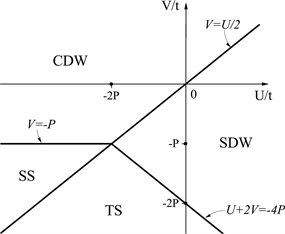(a) P > 0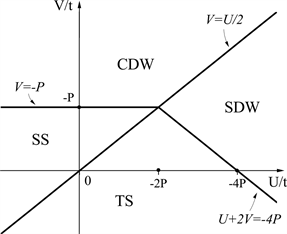(b) P < 0

Figure 1. The phase diagram of t-U-V-P model

4. 量子仿真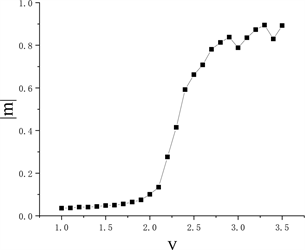Figure 2. The rise of $|m|$ in the t-U-V modelFigure 3. The distribution of $|m|$ in t-U-V-P model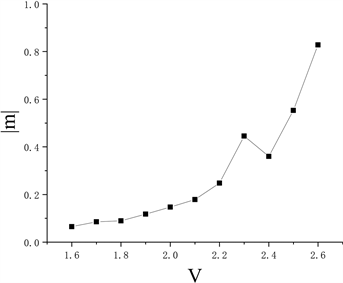Figure 4. The rise of $|m|$ in the t-U-V-P model

5. 结论(a) CDW: U = 0, V = 0, P = −2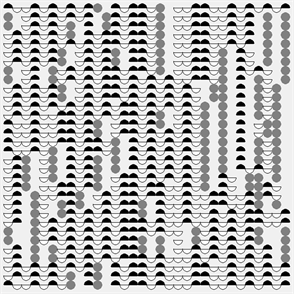(b) SDW: U =7, V = 3, P = −2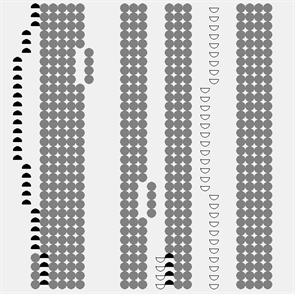(c) SS: U = −2, V = 1, P = −2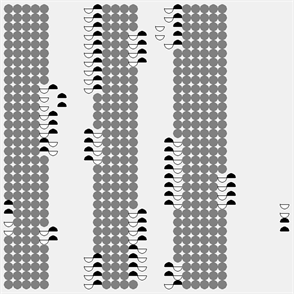(d) TS: U = 4, V = 0, P = −2

Figure S1. The characteristics of different states

NOTES

*通讯作者。

  Yu, J. and Ding H.Q. (2023) Phase Diagram of a Generalized Penson-Kolb-Hubbard Chain with the Occupa-tion-Dependent Hopping. Results in Physics, 44, Article ID: 106169. https://doi.org/10.1016/j.rinp.2022.106169  Baykusheva, D.R., Kalthoff, M.H., Hofmann, D., et al. (2023) Witnessing Nonequilibrium Entanglement Dynamics in a Strongly Correlated Fermionic Chain. Physical Review Letters, 130, Article ID: 106902. https://doi.org/10.1103/PhysRevLett.130.106902  Kreisel, A., Andersen, B.M., Rømer, A.T., Eremin, I.M. and Lechermann, F. (2022) Superconducting Instabilities in Strongly Correlated Infinite-Layer Nickelates. Physical Review Letters, 129, Article ID: 077002. https://doi.org/10.1103/PhysRevLett.129.077002  Murakami, Y., Takayoshi, S., Kanekoet, T., Läuchli, A.M. and Werner, P. (2023) Spin, Charge, and η-Spin Separation in One-Dimensional Photodoped Mott Insulators. Physical Review Letters, 130, Article ID: 106501. https://doi.org/10.1103/PhysRevLett.130.106501  Shao, C., Lu, S.T., Yu, C., Tohyama, T. and Lu, R. (2022) High-Harmonic Generation Approaching the Quantum Critical Point of Strongly Correlated Systems. Phys-ical Review Letters, 128, Article ID: 047401. https://doi.org/10.1103/PhysRevLett.128.047401  Ni, K.K., Ospelkaus, S., De Miranda, M.H.G., et al. (2008) A High Phase-Space-Density Gas of Polar Molecules. Science, 322, 231-235. https://doi.org/10.1126/science.1163861  Lu, M., Burdick, N.Q. and Lev, B.L. (2012) Quantum Degener-ate Dipolar Fermi Gas. Physical Review Letters, 108, Article ID: 215301. https://doi.org/10.1103/PhysRevLett.108.215301  Wu, C.H., Park, J.W., Ahmadi, P., Will, S. and Zwier-lein, M.W. (2012) Ultracold Fermionic Feshbach Molecules of 23Na40K. Physical Review Letters, 109, Article ID: 085301. https://doi.org/10.1103/PhysRevLett.109.085301  Sowiński, T., Dutta, O., Hauke, P., Ta-gliacozzo, L. and Lewenstein, M. (2012) Dipolar Molecules in Optical Lattices. Physical Review Letters, 108, Article ID: 115301. https://doi.org/10.1103/PhysRevLett.108.115301  Greif, D., Tarruell, L., Uehlinger, T., Jördens, R. and Esslinger, T. (2011) Probing Nearest-Neighbor Correlations of Ultracold Fermions in an Optical Lattice. Physical Review Letters, 106, Article ID: 145302. https://doi.org/10.1103/PhysRevLett.106.145302  Köhl, M., Moritz, H., Stöferle, T., Günter, K. and Ess-linger, T. (2005) Fermionic Atoms in a Three Dimensional Optical Lattice: Observing Fermi Surfaces, Dynamics, and Interactions. Physical Review Letters, 94, Article ID: 080403. https://doi.org/10.1103/PhysRevLett.94.080403  Lewenstein, M., Sanpera, A., Ahufinger, V., Damski, B., Sen, A. and Sen, U. (2007) Ultracold Atomic Gases in optIcal Lattices: Mimicking Condensed Matter Physics and Beyond. Advances in Physics, 56, 243-379. https://doi.org/10.1080/00018730701223200  Han, J. (2010) Direct Evidence of Three-Body Interactions in a Cold 85Rb Rydberg Gas. Physical Review A, 82, Article ID: 052501. https://doi.org/10.1103/PhysRevA.82.052501  Johnson, P.R., Tiesinga, E., Porto, J.V. and Williams, C.J. (2009) Effective Three-Body Interactions of Neutral Bosons in Optical Lattices. New Journal of Physics, 11, Article ID: 093022. https://doi.org/10.1088/1367-2630/11/9/093022  Büchler, H.P., Micheli, A. and Zoller, P. (2007) Three-Body Interactions with Cold Polar Molecules. Nature Physics, 3, 726-731. https://doi.org/10.1038/nphys678  Hammer, H.W., Nogga, A. and Schwenk, A. (2013) Colloquium: Three-Body Forces: From Cold Atoms to Nuclei. Reviews of Modern Physics, 85, Article No. 197. https://doi.org/10.1103/RevModPhys.85.197  Van Dongen, P.G.J. (1994) Extended Hubbard Model at Strong Coupling. Physical Review B, 49, Article No. 7904. https://doi.org/10.1103/PhysRevB.49.7904  Voit, J. (1992) Phase Diagram and Correlation Functions of the Half-Filled Extended Hubbard Model in One Dimension. Physical Review B, 45, Article No. 4027. https://doi.org/10.1103/PhysRevB.45.4027  Aligia, A.A., Arrachea, L. and Gagliano, E.R. (1995) Phase Diagram of an Extended Hubbard Model with Correlated Hopping at Half Filling. Physical Review B, 51, Article ID: 13774. https://doi.org/10.1103/PhysRevB.51.13774  Aligia, A.A. and Arrachea, L. (1999) Triplet Super-conductivity in Quasi-One-Dimensional Systems. Physical Review B, 60, Article ID: 15332. https://doi.org/10.1103/PhysRevB.60.15332  De Boer, J., Korepin, V.E. and Schadschneider, A. (1995) η Pairing as a Mechanism of Superconductivity in Models of Strongly Correlated Electrons. Physical Review Letters, 74, Article No. 789. https://doi.org/10.1103/PhysRevLett.74.789  Gogolin, A.O., Nersesyan, A.A. and Tsvelik, A.M. (1998) Bosonization and Strongly Correlated Systems. Cambridge University Press, Cambridge.  Wiegmann, P.B. (1978) One-Dimensional Fermi System and Plane xy Model. Journal of Physics C: Solid State Physics, 11, Article No. 1583. https://doi.org/10.1088/0022-3719/11/8/019  Boyanovsky, D. (1989) Field-Theoretical Renormali-sation and Fixed-Point Structure of a Generalised Coulomb Gas. Journal of Physics A: Mathematical and General, 22, Article No. 2601. https://doi.org/10.1088/0305-4470/22/13/051  Von Delft, J. and Schoeller, H. (1998) Bosonization for Beginners—Refermionization for Experts. Annalen der Physik, 7, 225-305. https://doi.org/10.1002/andp.19985100401  Voit, J. (1995) One-Dimensional Fermi Liquids. Reports on Progress in Physics, 58, Article No. 977. https://doi.org/10.1088/0034-4885/58/9/002  Xu, Y. and Ding H.Q. (2022) Ground-State Properties of the One-Dimensional Modified Penson-Kolb-Hubbard Model. Results in Physics, 41, Article ID: 105921. https://doi.org/10.1016/j.rinp.2022.105921  Aligia, A.A., Gagliano, E., Arrachea, L. and Hallberg, K. (1998) Superconductivity with s and p Symmetries in an Extended Hubbard Model with Correlated Hopping. The European Physical Journal B—Condensed Matter and Complex Systems, 5, 371-378. https://doi.org/10.1007/s100510050456  Dolcini, F. and Montorsi, A. (2013) Quantum Phases of One-Dimensional Hubbard Models with Three- and Four-Body Couplings. Physical Review B, 88, Article ID: 115115. https://doi.org/10.1103/PhysRevB.88.115115  Japaridze, G.I. and Kampf, A.P. (1999) Weak-Coupling Phase Diagram of the Extended Hubbard Model with Correlated-Hopping Interaction. Physical Review B, 59, Article No. 12822. https://doi.org/10.1103/PhysRevB.59.12822  Chen, W.T., Ding H.Q. and Zhang J. (2022) Theoretical Investigation of Four-Body Interaction in the One-Dimensional Extended Hubbard Model. Results in Physics, 34, Article ID: 105250. https://doi.org/10.1016/j.rinp.2022.105250  Xu, Y., Chen, W.T. and Ding H.Q. (2019) Coexistence of Superconductivity and Density Wave Orders in a One-Dimensional Correlated System. Chinese Journal of Physics, 67, 222-229. https://doi.org/10.1016/j.cjph.2020.07.006  Lloyd, S. (1996) Universal Quantum Simulators. Science, 273, 1073-1078. https://doi.org/10.1126/science.273.5278.1073  Georgescu, I.M., Ashhab, S. and Nori, F. (2014) Quantum Simulation. Reviews of Modern Physics, 86, Article No. 153. https://doi.org/10.1103/RevModPhys.86.153  Suzuki, M. (1976) Relationship between d-Dimensional Quantal Spin Systems and (d + 1)-Dimensional Ising Systems: Equivalence, Critical Exponents and Systematic Ap-proximants of the Partition Function and Spin Correlations. Progress of Theoretical Physics, 56, 1454-1469. https://doi.org/10.1143/PTP.56.1454  Suzuki, M., Miyashita, S. and Kuroda, A. (1977) Monte Carlo Sim-ulation of Quantum Spin Systems. I. Progress of Theoretical Physics, 58, 1377-1387. https://doi.org/10.1143/PTP.58.1377  Barma, M. and Shastry, B.S. (1978) Classical Equivalents of One-Dimensional Quantum-Mechanical Systems. Physical Review B, 18, Article No. 3351. https://doi.org/10.1103/PhysRevB.18.3351  Cullen, J.J. and Landau, D.P. (1983) Monte Carlo Studies of One-Dimensional Quantum Heisenberg and XY Models. Physical Review B, 27, Article No. 297. https://doi.org/10.1103/PhysRevB.27.297  Handscomb, D.C. (1964) A Monte Carlo Method Applied to the Heisenberg Ferromagnet. Mathematical Proceedings of the Cambridge Philosophical Society, 60, 115-122. https://doi.org/10.1017/S030500410003752X  Hirsch, J.E., Sugar, R.L., Scalapino, D.J. and Blankenbe-cler, R. (1982) Monte Carlo Simulations of One-Dimensional Fermion Systems. Physical Review B, 26, Article No. 5033. https://doi.org/10.1103/PhysRevB.26.5033  Blankenbecler, R., Scalapino, D.J. and Sugar, R.L. (1981) Monte Carlo Calculations of Coupled Boson-Fermion Systems. I. Physical Review D, 24, Article No. 2278. https://doi.org/10.1103/PhysRevD.24.2278  Hirsch, J.E. (1985) Two-Dimensional Hubbard Model: Nu-merical Simulation Study. Physical Review B, 31, Article No. 4403. https://doi.org/10.1103/PhysRevB.31.4403  White, S.R., Scalapino, D.J., Sugar, R.L., Loh, E.Y., Guber-natis, J.E. and Scalettar, R.T. (1989) Numerical Study of the Two-Dimensional Hubbard Model. Physical Review B, 40, Article No. 506. https://doi.org/10.1103/PhysRevB.40.506  Hirsch, J.E. (1984) Charge-Density-Wave to Spin-Density-Wave Transition in the Extended Hubbard Model. Physical Review Letters, 53, Article No. 2327. https://doi.org/10.1103/PhysRevLett.53.2327